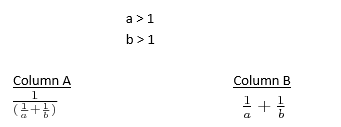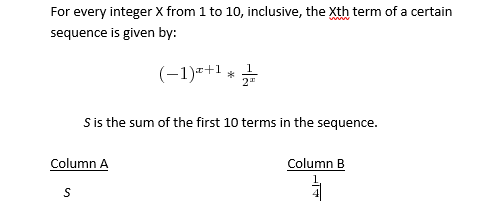+91-22-62437100 / 01 | help@reachivy.com
// //

GRE Quantitative Comparisons Quiz 2

Posted on Oct 15, 2017

To begin click Next

Question 1:

Working at constant rates, machine D completely makes b rolls of steel in 25 minutes and machine E completely makes b rolls of steel in one hour (b > 0).

Column A                              Column B
The number of                         The number of
rolls of steel                             rolls of steel made
made by machine                    by machine E
D in 2 hours and                      in 4 hours.
5 mins.
Question 2:

Column A                            Column B
The cost of b plums            The cost of c
at a cost of                         persimmons at a
c + 3 cents a piece              cost of b + 3 cents a piece
Question 3:
|y|-1 < -y

Column 1                             Column 2

y                                                 |y|

Question 4:

E and F are the midpoints of the opposite sides of square ABCD. The distance between one of the midpoints and any of its opposite vertices is square root of ‘10’

Column X                                Column Y
The area of region ABCD               7
Question 5:

Set A: {1, 2, 4, 8, 16, 32}
Set B: {all prime numbers less than 10}

Column A                                          Column B
The number of distinct integers           The number of integers
included in A U B divided by 6.             in A B
Question 6:

Column A                                                       Column B
The standard deviation of the sample               The standard deviation of the sample
measurements 0, 1, 2, 4, and 8.                         measurements 1, 2, 3, 5, and 9.
Question 7:
Chase's salary, which is greater than \$20,000, is 80 percent of Katy's salary. Timan's salary is 80% of Chase's salary.

Column A                                      Column B
Katy's salary                                 Timan's salary
Question 8:Question 9:You have answered all the questions in the quiz. Press Submit to view your score